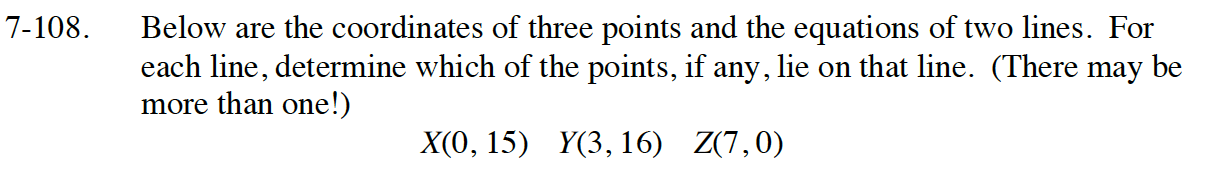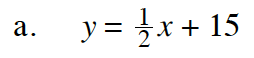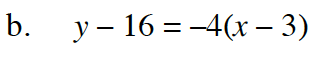Home > CC4 > Chapter 7 > Lesson 7.2.3 > Problem7-108

7-108.
1. Below are the coordinates of three points and the equations of two lines. For each line, determine which of the points, if any, lie on that line. (There may be more than one!) Homework Help ✎

2. X(0, 15) Y(3, 16) Z(7, 0)

1. y =x + 15

2. y – 16 = –4(x – 3)Individually check to see if X, Y, and Z are on the line.
Do this by substituting the given coordinates into the original equation.
There may be more than one point correct!

$\text{True or false: }15 = \frac{1}{2}(0) + 15$

True: X is on the line.

$\text{True or false: }16 = \frac{1}{2}(3) + 15$

False: Y is not on the line.

$\text{True or false: }0 = \frac{1}{2}(7) + 15$

False: Z is not on the line.Follow the same procedure in part (a).